### Home > CC2 > Chapter Ch8 > Lesson 8.3.1 > Problem8-62

8-62.
1. Simplify each expression. Homework Help ✎

2.  a.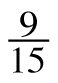÷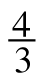b. −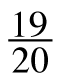+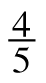c. −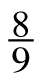÷ (−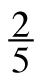) d. 3÷ 1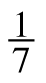e. −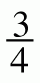− (−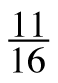) f.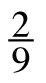·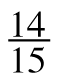· (−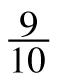) g. −10+ (−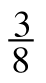) h.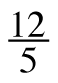÷(−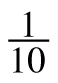)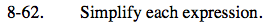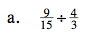When you divide by a fraction, you are multiplying by its reciprocal.

$\frac{9}{20}$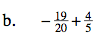Find a common denominator.

$-\frac{3}{20}$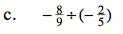See part (a).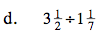Convert these mixed numbers into fractions greater than one.

$\frac{49}{16}$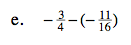$\text{This is the same as }-\frac{3}{4}+\frac{11}{16}.$

$-\frac{1}{16}$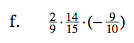$\frac{2}{9}\Big(-\frac{9}{10}\Big)\cdot\frac{14}{15}$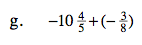You may add whole numbers and fractions separately.

$(-10)+\left(-\frac{4}{5}+-\frac{3}{8}\right)$

$-11\frac{7}{40}$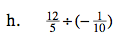See part (a).